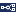FAQ: How can I compute and use visual angles in Experiment Builder?Jono Research Support SpecialistJoined: Sep 2020 Posts: 222 02-15-2022, 09:40 AM Summary For some tasks, it may be desirable to specify the location of stimuli in degrees of visual angle rather than in pixels. For example in a simple prosaccade task, targets should appear at +/- 5 and 10 degrees from the screen center. In Experiment Builder, it is possible to convert degrees of visual angle to a pixel distance value using math functions available in Python. You can then set the location of the stimuli based on the calculated pixel value. This thread outlines the basic equation, the required variables and measurements and how to apply the math functions. The Experiment Builder example (attached below as a packaged project) illustrates how to position a target at 4, 8 and 12 degrees to the left and right of the screen centre. Visual Angle to Pixel Equation The math underlying the equation below is detailed more fully on this visual angle blog post on our website.Experiment Builder Implementation In order to use this formula to convert from degrees of visual angle to pixels, we need to know the screen dimensions (just horizontal in this case) and distance. These values (in mm) are entered in two variables that have been added to the Experiment sequence.We also need to know the degree value to be converted into pixels. In other words, we need to know how far from the screen center the target should be displayed. This differs for each trial, so is provided by a column in the data source attached to the Trial Sequence.Finally, we need the horizontal pixel resolution of the screen. Experiment Builder can access this value via the project's DISPLAY.width property. The equation is calculated for each trial in the Update Attribute node labelled CALCULATE_POSITION, which updates the value of the Step_Size_Pix variable.Calculating the Distance and Updating the Stimuli Location Within the CALCULATE_POSITION Update Attribute, two attributes are updated. The first ‘Step_Size_Px’ is updated with the degree to pixel formula, shown belowThe second attribute is the location of the stimuli called ‘Square’ - a small red rectangular resource. This x-axis location is updated as the screen centre + the step size pixel value. If the step size pix value is negative the x-axis value is to the left (a smaller pixel value), if positive it is to the right of the center (a larger pixel value). Attached Example In the attached example there are six trials, with a 4, 8 and 12-degree step to the left and the right. Each trial begins with a central black dot on the screen for 500ms, followed by a display screen showing an offset red square at the values calculated by the formula. Please download and take a look at this example and don’t hesitate to contact support@sr-research.com with any questions. Attached FilesVisual_Angle_Calculation.ebz (Size: 30.35 KB / Downloads: 13)